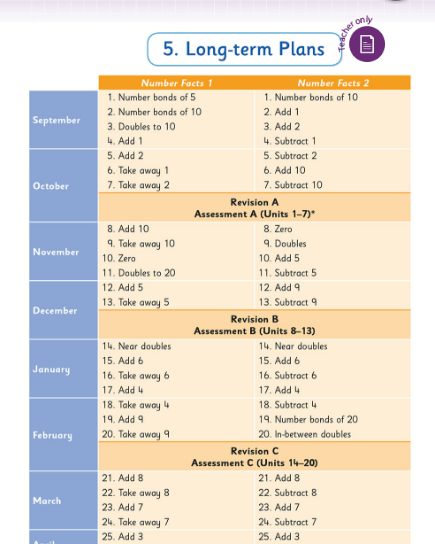# Monthly Archives: September 2018

## Maths by Month – October (updated 2018)

Category : Uncategorized

Welcome to the second installment in this year’s series of posts designed to explore the Operation Maths topics on a month-by-month basis, giving teachers greater insights into the concepts at hand, when they are most relevant. While each monthly overview will specifically zone in on the Operation Maths topics for that particular month, the information and suggestions will be relevant to ALL primary teachers, whether they are Operation Maths users or not.

HINT: To ensure you don’t miss out on any future Maths by Month blog-posts, please subscribe to the Operation Maths blog via email, on the top right hand of this page.
Another way to keep up to date an all new maths-related developments is to like/follow the Edco Primary Maths page on Facebook and/or Twitter

### Operation Maths for Junior Infants to Sixth Class:

• Operation Maths users can also access a class specific, month-by-month list of relevant links and online resources via the Weblinks document, accessible on www.edcolearning.ie.
2. Click on the At School Book/Pupil’s Book for your class level.
3. Click on the Edco Resources icon (on book cover image on left-hand side)
• Also accessible on  www.edcolearning.ie.  are the custom-made digital resources to support these topics. These will all be viewable when you click on the Edco Resources icon as directed above.

HINT: If you are new to Operation Maths this year or have changed class level, be sure to check out the Quick Start Guide to the Operation Maths books and the companion Quick Start Guide to the Operation Maths Digital Resources
Don’t forget that Operation Maths also has you covered for planning whether you’re teaching a single class or multi-class.

### Other suggestions for October:

• The October plan for third to sixth classes has deliberately allowed for a free week, to enable teachers to engage with Maths Week, held every year at this time. This year, Maths Week will run from 13-21 October. Make sure to register your school at the link above and then organise some fun maths activities for your class or school. You can follow the links in the site to find out more about Maths mazes, Maths Art (which, coincidentally, links very well with October Operation Maths for 3rd and 4th classes i.e. tessellations in 2D shapes), Maths and historycode breaking and lots more.
• You could also make Maths Week become a game-themed week in your class. Teachers of third to sixth classes could use the Games Bank in the Operation Maths TRB. Teachers of infants to second classes can use any of the games listed in the short-term plans in their TRBs.
• Another option for Maths Week, if you didn’t already do it in September,  is Jo Boaler’s  Week of Inspirational Maths. Click on the link for an overview of the activities in Week of Inspirational Math, and scroll down to the bottom of the page to access all the resources; K-2 roughly align with Infants to 2nd and Grades 3-5 roughly align with 3rd-6th classes.

We’re here to help!
If you have any questions on Operation Maths, Number Facts or anything related to primary maths over the course of the school year, please PM or contact Edco Primary Maths via Facebook and/or Twitter

## Digging Deeper into … Addition and Subtraction (infants to second class)

Category : Uncategorized

For practical suggestions for families, and links to useful digital resources, to support children learning about the topic of addition and subtraction, please check out the following post: Dear Family, your Operation Maths Guide to Addition and Subtraction

A quick look at the maths curriculum for junior and senior infants will reveal that, within the strand of number, there are no strand units entitled operations, addition or subtraction, as are evident in the curriculum for first and second classes. However both operations are there – under the guise of combining, partitioning and comparing.

Addition and subtraction are two of the four basic mathematical operations (multiplication and division being the other two):

• Addition involves the joining/combining of two or more quantities/sets/parts to get one quantity/whole/set, typically referred to as the sum or total. There are two main types: active (2 children at a table and 3 more join them) or static (2 boys and 3 girls at a table, how many children in all?)
• There are three types of subtraction:
• take-away (active) which involves the removal/deduction of one quantity/part from a whole amount/quantity
• comparison (static) which involves identifying by how much one quantity/set is more or less than another (the difference)
• missing addend (active) which involves identifying the amount needed to combine with a known part to make a whole.

In each type of subtraction we know the total/whole and a part and we need to find the missing part, which could be the amount left, the difference or the missing addend.

The types of addition and subtraction are explained very clearly and succinctly in the Origo One videos below.

### Relationship between addition and subtraction

As shown in the videos above, addition and subtraction are inverse operations; we can demonstrate addition by adding more to an existing amount; the reverse action would involve removing an amount, thus demonstrating subtraction as take away. In contrast to traditional maths schemes, which often have separate chapters for each of these operations, Operation Maths predominantly teaches addition and subtraction together, as related concepts. Teaching the operations in this way will encourage the children to begin to recognise the relationships between addition and subtraction.

Beginning in first and second classes, the children are enabled to understand addition and subtraction as being the inverse of each other, which will progress towards using the inverse operation to check calculations in higher classes.

### CPA Approach within a context

As mentioned repeatedly in previous posts, both the Operation Maths and Number Facts series are based on a CPA approach. Furthermore, as was referenced in the videos above, for the children to develop a deep understanding of the different types of addition and subtraction, there has to be some context or story, with which they can identify. This, in turn, should be explored via progression through concrete, pictorial and abstract stages.

This context can be simply made up by the teacher or be inspired by a picture book that the class is reading. It can be modeled using the concrete materials available in the classroom (eg plastic animals, toy cars, play food etc. ) and/or using the Operation Maths Sorting eManipulative (see below) and the extensive suite of inbuilt images; the images can be shown either with or without a background (background options include five and ten frames, set outlines and various grids).HINT: To find out more about how to use the 5, 10 and 20 frames that accompany the Operation Maths series please read on here: http://operationmaths.ie/youve-been-framed-closer-look-ten-frames/

As the children progress, the need arises to record the operations using some graphic means. Initially, this can include representing each of the items in the story with counters and/or cubes. In turn, bar models could also be used to represent number relationships, while bearing in mind that different types of bar models will be required to model different context and types of subtraction (even though the subtraction sentences, if using them, might look exactly the same). Using the examples below, the first bar model (a part-whole bar model) could be used to model this story: Snow White had seven dwarfs. If four of these went to work, how many were left at home? Whereas, the second bar model (a comparison bar model) would better suit this story: the seven dwarfs all wanted to sit down at the table but there were only four chairs. How many dwarfs had no chair?While bar models do not specifically appear until in the pupils books until Operation Maths 3, the children could use and explore simple bar models. Thinking Blocks Jr is based on simple bar models and could be shown to the class on the IWB while the children suggest answers and labels on their Operation Maths MWBs.  Then the children could draw simple models in their books to help solve the word problems there. Furthermore , as shown above, the Bar Modelling eManipulative could also be used to create bars of different length.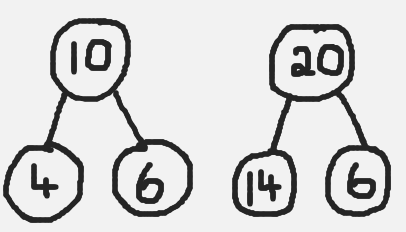Before rushing too quickly into abstract recording (using only digits and symbols), an alternative intermediary stage could be to represent the relationships, using a branching bond (opposite). Similar to the part-whole bar model earlier, this branching bond structure encourages the children to appreciate that two sets/parts ( 4 and 6) can be combined to make a larger set/whole (10). Inversely, when a part (4) is removed from the whole (10), a part is left (6). This bond structure can also represent the missing addend type of subtraction: if a part was hidden (6), the question could be asked  what must be added to 4 to make 10.

Both branching bonds and simple bar models are used throughout the Number Facts series to represent relationships and demonstrate strategies. They are also used throughout the Operation Maths 3-6 books, but in increasingly more complex situations.

### The meaning of the equals sign

With the formal introduction of addition number sentences in senior infants (ie the recording of relationships using the plus and equals sign), followed by the formal introduction of subtraction sentences (using the minus sign) in first class, comes the need to correctly interpret the purpose of the equals sign as identifying equivalence; ie that the value on one side of the equals sign is the same as the value on the other side. It is essential at this stage that the children don’t interpret the equals signal incorrectly as being a signpost indicating that the answer is coming next. A pan or bucket balance is an extremely valuable resource to help demonstrate equivalency, as can be seen in the video below.

Calculations in the Operation Maths book are often shown vertically and horizontally. When presented horizontally, it is often misinterpreted that the children must now rewrite the calculation vertically, to be solved using the traditional column method (see more on the column method in the next section). Rather, presenting calculations horizontally is a deliberate effort to encourage the children to explore how to solve the calculation using a concrete based approach and/or using a mental strategy, as opposed to always tackling these calculations in a written way.

### Looking at more complex numbers

In first and second classes, once introduced to operations using two-digit numbers, children can often have tunnel vision (or column vision) regarding addition and subtraction calculations: they “do” the units, and then the tens, without really looking at the whole numbers or the processes involved.

One way in which you can encourage the children to look at and understand these operations better is by using a CPA approach. This means that the children’s initial experiences should involve groupable base ten concrete materials (e.g. bundling straws or lollipop sticks, ten-frames and counters, unifix or multi-link cubes arranges in sticks of ten, see below), where a ten can be physically decomposed  into ten units and vice versa, before moving on to pregrouped base ten materials (eg base ten blocks/Dienes blocks, base ten money and/or Operation Maths place value discs) which require a swap to exchange a ten for ten units and vice versa.When children are comfortable with the manipulating the concrete materials, they can move on to a process whereby these materials are represented pictorially and/or demonstrate the process using a suitable the visual structure eg an empty number line and/or bar model. Abstract exercises, where the focus is primarily on numbers and/or digits, should only appear as part of the final stage of this process.When exchanging tens and units (or tens and hundreds in second class), reinforce that a ten is also the same as 10 units, and that a hundred is the same as 10 tens and is the same as 100 units. The use of non-canonical arrangements of numbers (e.g. representing 145 as 1H 3T 15U or  14T 5U), as mentioned in Place Value, can also be very useful to children as they develop their ability to visualise the regrouping/renaming process. The Operation Maths Place Value eManipulative, accessible on edcolearning.ie,  is an excellent way to illustrate this and explore the operations in a visual way.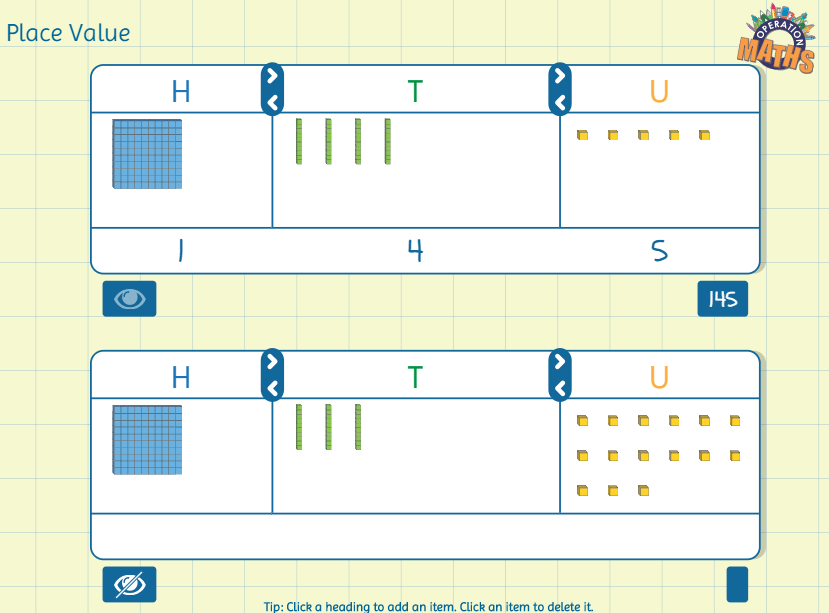### Mental strategies are as important as written methods

In first and second classes, the traditional, written algorithms for addition and subtraction, i.e. the column methods, are important aspects of these operations. However, in real-life maths, mental calculations are often more relevant than written methods. Also, as mentioned previously, children can often have tunnel vision (or column vision) regarding addition and subtraction calculations; they ‘do’ the units, then the tens, without really looking at the entire numbers or the processes involved. Therefore, while the column method for addition and subtraction is an important aspect of this topic, equally important is the development of mental calculation skills, via a thinking strategies approach.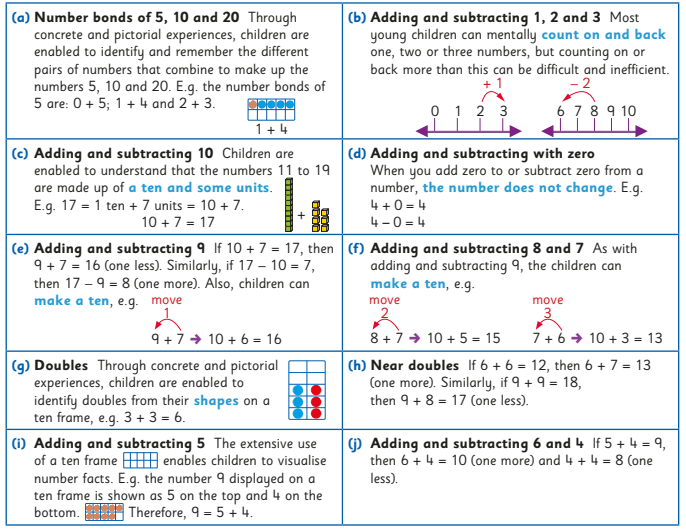From Number Facts 1 & 2

Thus, one of the main purposes of the operation chapters in Operation Maths is to extend the range of strategies that the children have and to enable them to apply the strategies to numbers of greater complexity i.e. for the children to become efficient and flexible, as well as accurate. As the same calculation can often be done mentally in many different ways, the children have to develop their decision-making skills so as to be in a position to decide what is the most efficient strategy to use in each situation.When meeting new calculations, ask the children, as often as possible, can they do it mentally, and how, so that they become increasingly aware of a range of mental calculation skills and approaches. In this way the children will also be developing their decision-making skills, so as to be in a position to decide the most efficient strategy/approach to use.

HINT: Number Talks are a fabulous resource to use alongside the Operation Maths and/or Number Facts series, as they complement their thinking strategies approach. Read on here to find out more about where both Operation Maths and Number Talks overlap.

### Key messages:

• There are different types of addition and subtraction and children need to explore the different types to gain a deep understanding of the concepts
• As children encounter new numbers and new number ranges, be it numbers to ten in infants, teen numbers to 199 in first and second classes, they should be afforded ample opportunities to combine to make these amounts, partition these amounts and compare these amounts using concrete materials and via some story-like context.
• Initial recording of these relationships should be via counters and cubes etc, before moving on to pictorial representations of the same and/or using frames, maths rack, bar models, branching bonds etc.
• Addition and subtraction number sentences, that use only digits and symbols, should be avoided until the children demonstrate readiness for this more abstract stage.
• Encourage the children to use and develop mental strategies and avoid focussing almost exclusively on the formal, traditional ways of doing addition and subtraction ie column method.

This short video from Graham Fletcher showing the progression of addition and subtraction from the infant classes to the formal written algorithm, with three and four-digit numbers, is very worthwhile viewing and summarises the key messages well.

• Dear Family, your Operation Maths Guide to Addition and Subtraction includes practical suggestions for supporting children, and links to a huge suite of digital resources, organised according to class level.
• Operation Maths Digital Resources: As always don’t forget to access the linked digital activities on the digital version of the Pupil’s book, available on edcolearning.ie. Tip: look at the footer on the first page of each chapter in the pupil’s book to get a synopsis of what digital resources are available/suggested to use with that particular chapter.
• For more hints and tips specific to each class level, check out the “What to look out for” section in the introduction to this topic in the Teacher’s Resource Book (TRB)
• Number Talks book by Sherry Parrish
• Mental Maths handbook for Addition and Subtraction from the PDST
• Splat! Similar to Number Talks, these free resources from Steve Wyborney encourage discussion and reasoning. Play the PowerPoint presentations on your class IWB while the children use their Operation Maths MWBs to respond.
• Addition & Subtraction Board on Pinterest

## Thinking Strategies for Addition and Subtraction Number Facts

Category : Uncategorized

### What are number facts?

Number facts are the basic number facts that, it is hoped, children could recall instantly, so as to improve their ability to compute mentally and use written algorithms. Traditionally referred to as tables, the addition and subtraction number facts typically include all the addition facts up to 10 + 10 and their inverse subtraction sentences.

Some of the big ideas about number facts:

• Some facts are easier than others to recall – which ones, do you think?
• The easier facts can be used to calculate other facts – which ones, do you think?
• The same fact can be calculated using various approaches – these approaches are often referred to as thinking strategies – see more below.
• Using thinking strategies means that the children can apply the understanding, to facts beyond the traditional limits of “tables”.

### What are thinking strategies?

A thinking strategy is a way to think about a process to arrive efficiently at an answer. For example, if asked to add 9 to a number, one could think of moving 1 from the other addend to the 9 so as to make a 10, which therefore becomes an easier calculation (see below)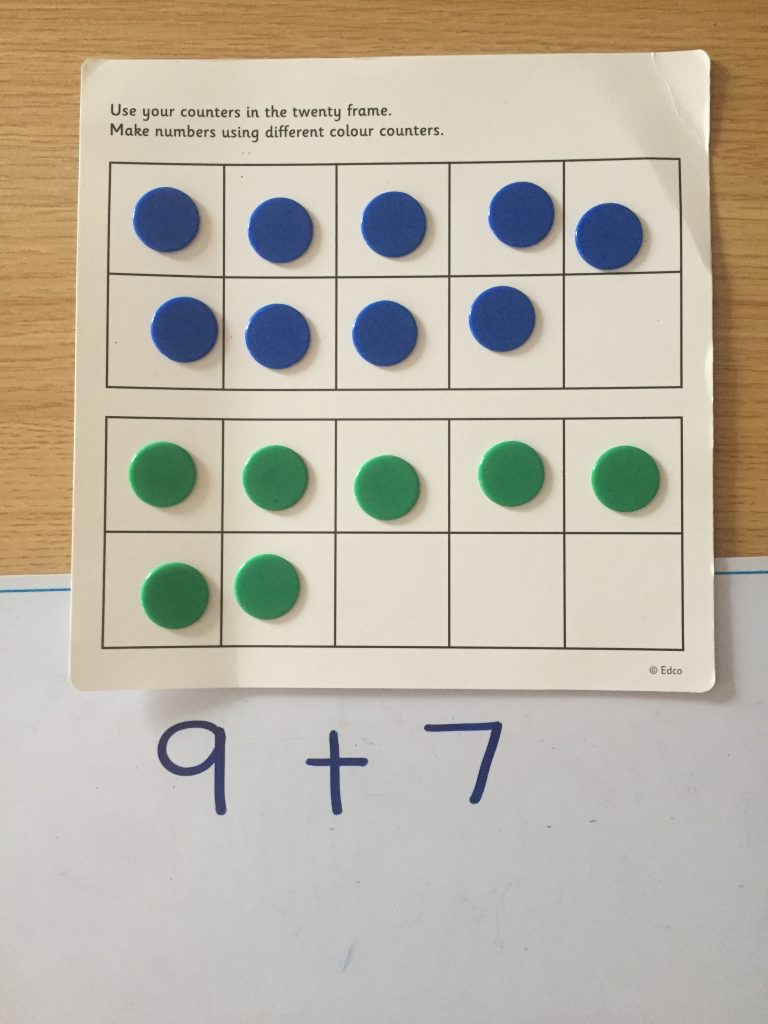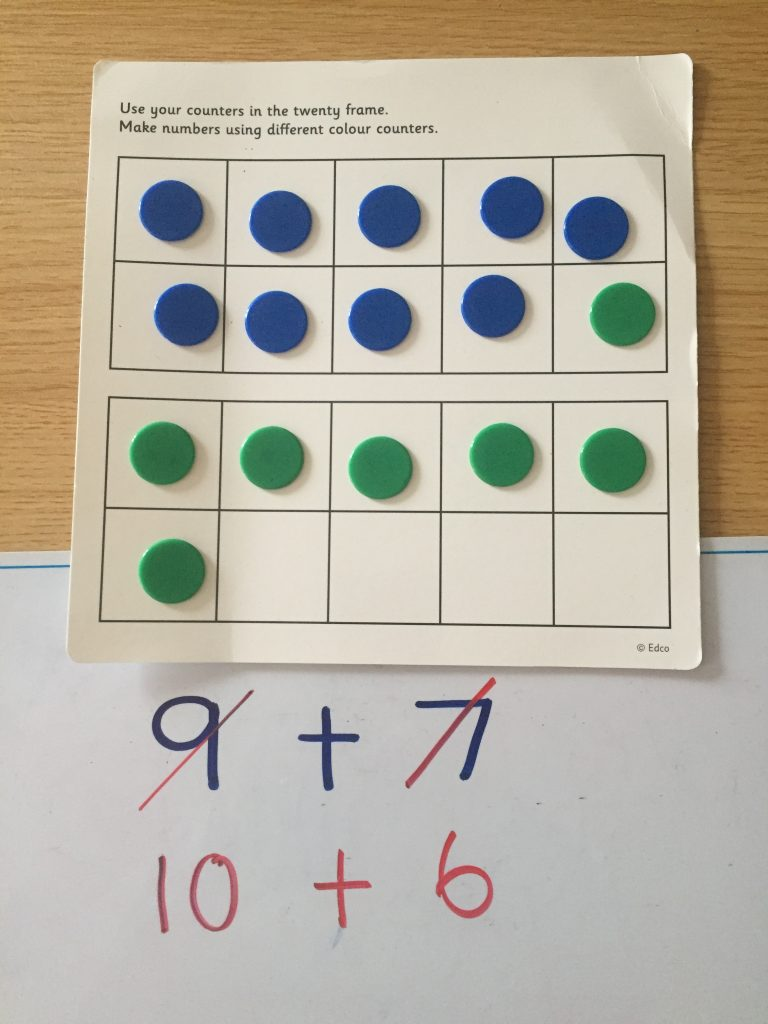The Operation Maths books for first and second classes emphasise three specific thinking strategies throughout: counting on from the biggest number, using doubles and near doubles and using the number bonds for ten (see image below). The doubles facts and bonds of ten are also included on the pull-out flap at the back cover to the pupils books, both for quick reference and to emphasise their importance.From Operation Maths 2 At School Book

In the case of doubles, near doubles and bonds of ten, these key sets of number facts tend to be easier for children to understand and recall. These facts also make up a core section of the total addition facts to 10 + 10, as highlighted below on the addition square. When these become known facts, they can then in turn be used to calculate unknown facts (eg if 7 + 3  = 10, then 7 + 4 = 11), thus covering an even greater number of the total addition facts.Furthermore, addition and subtraction are taught together throughout the Operation Maths series, so that, rather than compartmentalising each operation, the children develop a better understanding of how both concepts relate to each other. In this way, the basic subtraction facts are easier to acquire, as they are understood to be the inverse of the more familiar addition facts.

Traditionally, learning “tables” had been by rote, but current research suggests that this is ineffective for the majority of children. In contrast, children should be taught to visualise numbers and to use concrete materials, images and thinking strategies to use what they know to solve what they do not know. Below are examples of some useful thinking strategies for the basic addition and subtraction facts (taken from Number Facts 1 & 2, Edco, 2018)From Number Facts 1 & 2

There can often be different ways to think about the same fact (or groups of facts), and the children should always be encouraged both to identify alternative approaches and to choose their preferred strategy. For example:

8 + 6 = (5 + 3) + (5 + 1) = 10 + 4 (make a ten) = 14
8 + 6 = 10 + 4 (move 2 from 6 to 8 to make a ten) = 14
8 + 6 = 7 + 7 (move 1 from 8 to 6 to make a double) = 14

Once the children understand how to arrive at an answer via a thinking strategy, they can then apply this thinking strategy to more complex calculations that are beyond the traditional 10 + 10 ceiling of “tables”; for example if I understand different ways to calculate that 8 + 6 = 14, then I can use these ways to mentally calculate 18 + 6 , 18 + 16 etc.

### Computational Fluency:

‘Fluency requires the children to be accurate, efficient and flexible.’ (Russell, 2000).

The primary aim of both the Operation Maths and Number Facts series (see more information on Number Facts below) is to enable the children to become computationally fluent. To achieve computational fluency, the children must be accurate, efficient and flexible:

• Accurate: the children must arrive at the correct answer, e.g. 8 + 6 = 14.
• Efficient: the children must calculate the answer in an efficiently. A child who produces an answer of 14 in response to the question 8 + 6 by ‘counting all’ (eg have to count up to a total using using counters, fingers, etc.) may be accurate but is not efficient.
• Flexible: children must be able to visualise and mentally manipulate numbers in order to see how they might be broken down and recombined to get an accurate and efficient answer (as shown with the various ways to consider 8 + 6 above).

Thus, flexibility is the key to fluency. A child who only knows that 8 + 6 = 14 becasue they have memorized that fact, is missing out on all the various possible connections between those numbers, subsequently hampering future connection-building. In contrast, a child who is flexible with number facts is one with a well-developed number sense, who can see the connections both between and within numbers, i.e. they can partition and/or combine numbers into more compatible (friendly) amounts and can apply their strategies to numbers beyond those they have dealt with. Thus, a thinking strategies approach will not only be effective for aiding understanding and recall of the basic facts up to 10 + 10, a thinking strategies approach can enable children to apply these mental computation skills to numbers beyond this traditional ceiling e.g. 19+ 5, 29 + 6 etc (see below).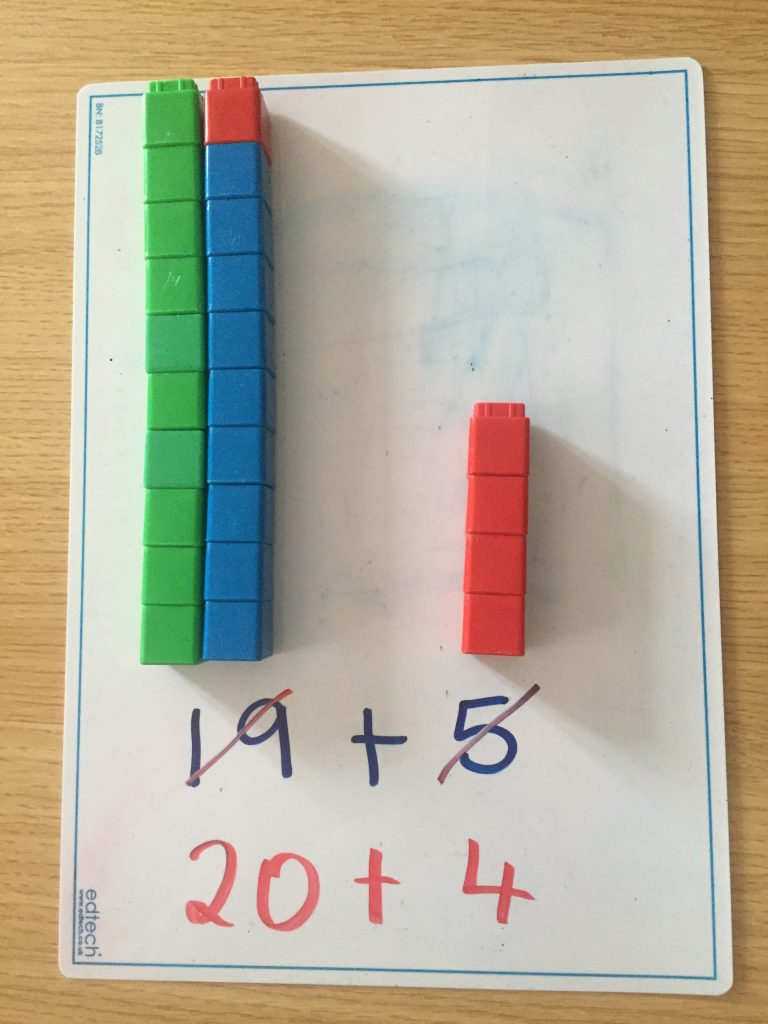### The Number Facts Series from Edco

Number Facts is latest addition to the Edco Primary Maths stable, and it is a series of activity books designed to foster fluency in number facts for primary school children from First Class. The series features an innovative approach to the acquisition of basic number facts, and, like Operation Maths, teaches children to understand, not just do, maths.In contrast to the more traditional drill-and-practice workbooks, which just test whether the answer is known, Number Facts teaches children to visualise numbers pictorially and to use these images and thinking strategies to become more adept at manipulating numbers. The specific focus of Number Facts will be to develop children’s thinking strategies and apply these to the basic number facts in such a way as to promote the child’s ability to visualise and recall these facts, thereby achieving fluency.

Both this rationale, and the suggested teaching approaches to the teaching of the basic addition and subtractions facts for first and second classes, are clearly outlined in the Teachers Resource Book (TRB) which accompanies the series, and which is downloadable here. This TRB also includes a Long Term Plan for both first and second classes (see extract below), outlining a logical progression for the various fact groups throughout the school year. To view sample pages from the pupils Number Facts books please click here. Sample copies of all the books are also available from your local Edco reps.